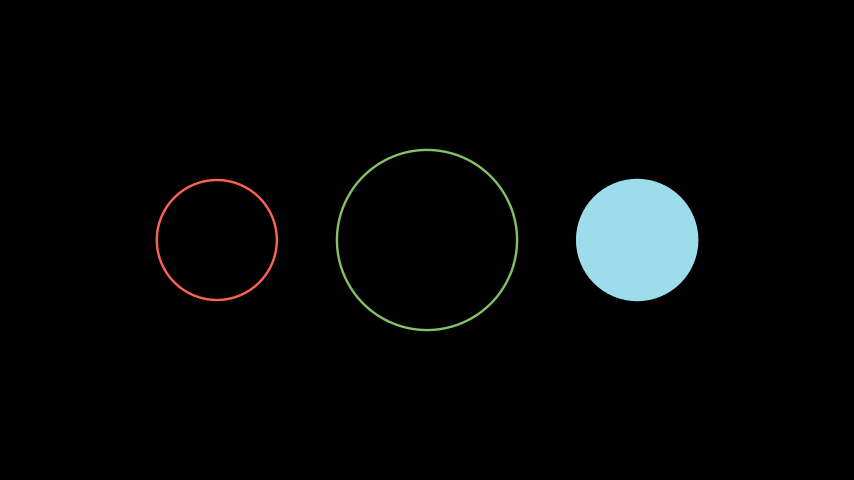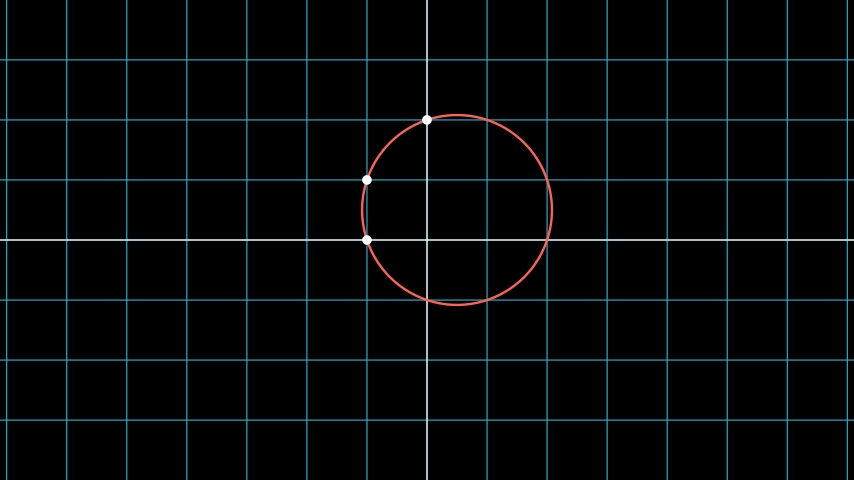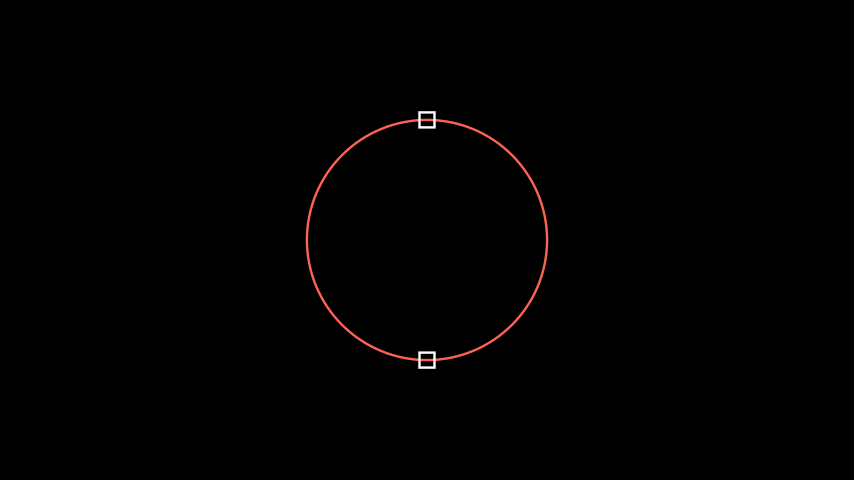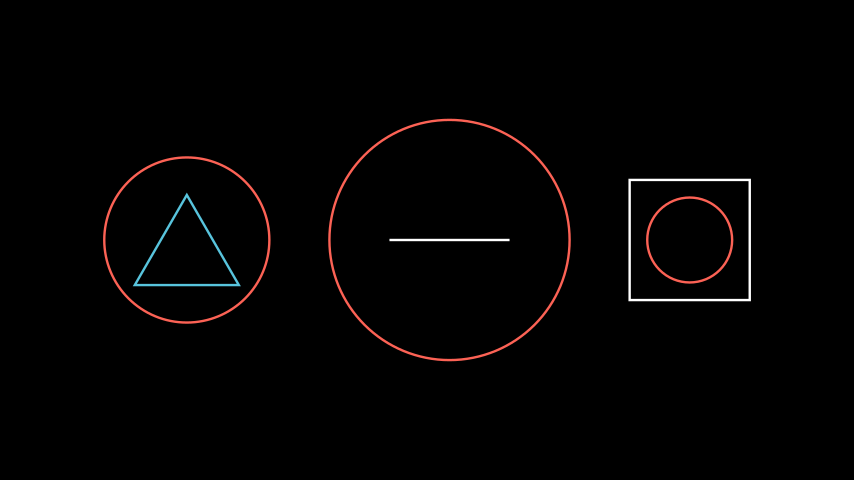# Circle¶

Qualified name: `manim.mobject.geometry.Circle`

A circle.

Parameters

Examples

Example: CircleExample```from manim import *

class CircleExample(Scene):
def construct(self):

circle_group = Group(circle_1, circle_2, circle_3).arrange(buff=1)
```

Methods

 `from_three_points` Returns a circle passing through the specified three points. `point_at_angle` Returns the position of a point on the circle. `surround` Modifies a circle so that it surrounds a given mobject.

Attributes

 `animate` Used to animate the application of a method. `animation_overrides` `depth` The depth of the mobject. `height` The height of the mobject. `width` The width of the mobject.
static from_three_points(p1, p2, p3, **kwargs)[source]

Returns a circle passing through the specified three points.

Example

Example: CircleFromPointsExample```from manim import *

class CircleFromPointsExample(Scene):
def construct(self):
circle = Circle.from_three_points(LEFT, LEFT + UP, UP * 2, color=RED)
dots = VGroup(
Dot(LEFT),
Dot(LEFT + UP),
Dot(UP * 2),
)
```
Parameters
• p1 (Sequence[float]) –

• p2 (Sequence[float]) –

• p3 (Sequence[float]) –

point_at_angle(angle)[source]

Returns the position of a point on the circle.

Parameters

angle (class: float) – The angle of the point along the circle in radians.

Examples

Example: PointAtAngleExample```from manim import *

class PointAtAngleExample(Scene):
def construct(self):
p1 = circle.point_at_angle(PI/2)
p2 = circle.point_at_angle(270*DEGREES)

s1 = Square(side_length=0.25).move_to(p1)
s2 = Square(side_length=0.25).move_to(p2)
```
Returns

The location of the point along the circle’s circumference.

Return type

`numpy.ndarray`

surround(mobject, dim_to_match=0, stretch=False, buffer_factor=1.2)[source]

Modifies a circle so that it surrounds a given mobject.

Parameters
• mobject (`Mobject`) – The mobject that the circle will be surrounding.

• dim_to_match (`int`, optional) –

• buffer_factor (`float`, optional) – Scales the circle with respect to the mobject. A buffer_factor < 1 makes the circle smaller than the mobject.

• stretch (`bool`, optional) – Stretches the circle to fit more tightly around the mobject. Note: Does not work with `Line`

Examples

Example: CircleSurround```from manim import *

class CircleSurround(Scene):
def construct(self):
triangle1 = Triangle()
circle1 = Circle().surround(triangle1)
group1 = Group(triangle1,circle1) # treat the two mobjects as one

line2 = Line()
circle2 = Circle().surround(line2, buffer_factor=2.0)
group2 = Group(line2,circle2)

# buffer_factor < 1, so the circle is smaller than the square
square3 = Square()
circle3 = Circle().surround(square3, buffer_factor=0.5)
group3 = Group(square3, circle3)

group = Group(group1, group2, group3).arrange(buff=1)